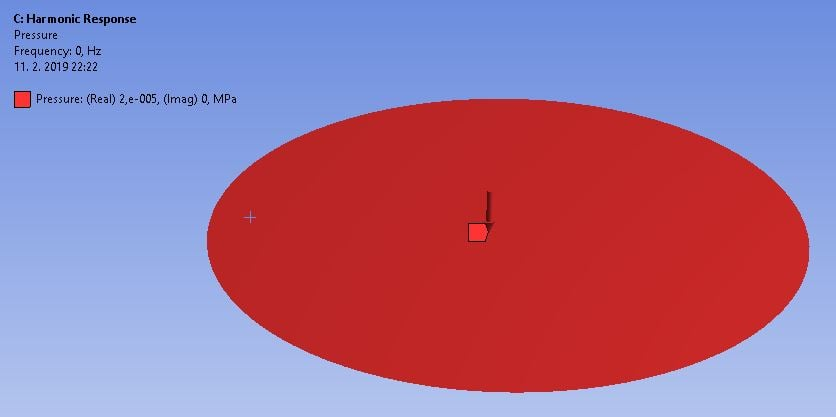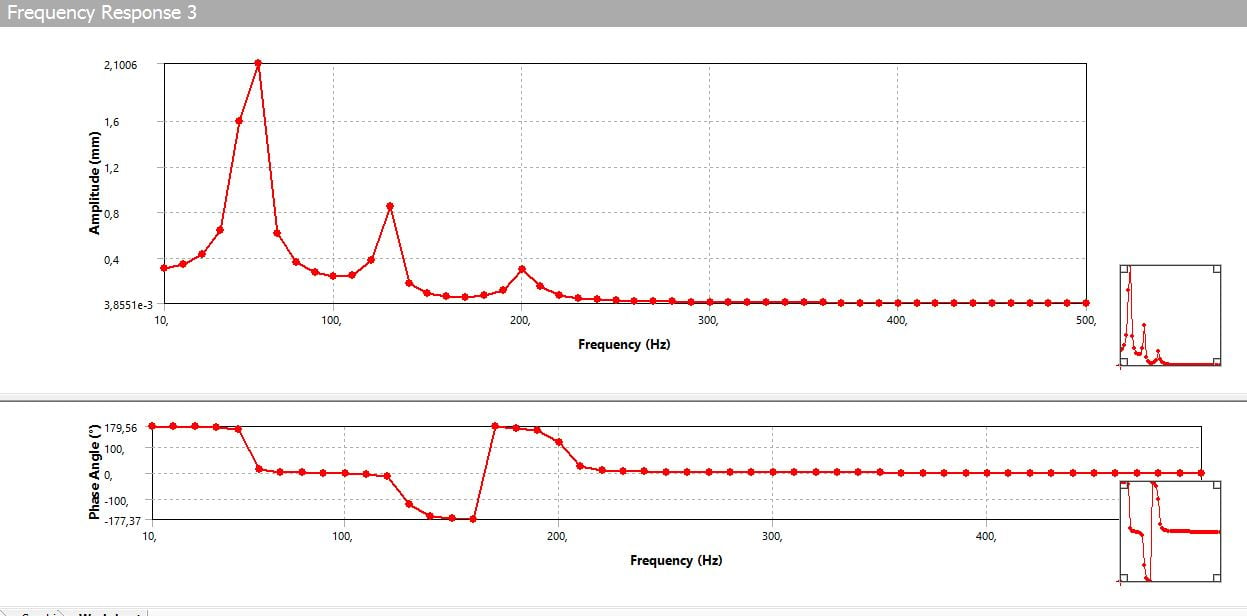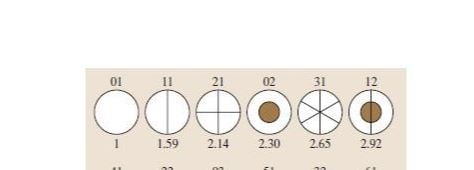# harmonic response analysis found only radially symmetric modes

Hello,

i have performed a modal analysis of prestressed ideal circular membrane (homogenous), clamped on its edges.

Modal analysis found following normal modes (in Hertz): 55,8; 89,06; 119,46; 128,45; 148,55; 163,46; 176,89; 198; and so on

Next i performed a harmonic analysis, because i wanted to know the displacement  at different frequencies.

The load was 20Pa pressure on whole area of membrane:Suprisingly, amplitude is critical only for RADIALLY SYMMETRIC modes: 55,58Hz; 128,45; 198HzWhy is that ?

Why only radially symmetric normal modes are critical?

By the way (just for explanation), the radially symetric mode from the following picture is 1st mode and 4th mode• edited February 2019

Well for the other modes (say11), the two halfs (about centre line) are in opposite phase. So this constant load does not have different phase (constant all over plate with same phase and amplitude) hence it can not excite it that much.

Easy to think about this is for a pinned-pinned beam. The first mode is half a sine (n =1), second is a full sine (n=2). If we have a constant load on the beam, then the anti-symmetric modes about the centre point of the beam (even multiples, that is n= 2, 4,..) can not be excited much since that would require a force that has opposite phase about the centre line. Thus here a symmetric load excites symmetric modes.

Same goes if we had a point force at the centre point that would not excite the anti-symmetric modes that have no movement there.

It is all maths, and it is a term in the solution that involves the inner product (integral not algebra), of the eigenmodes and the excitation. That will give how much a mode is excited. In the case of this point force one gets for the second mode (n=2), <sin(2pix/L), Dirac delta(x-L/2)>, that gives sin(2piL/2L) = sin(pi)=0, thus it can not be excited. One can do similar things for a plate, but that would involve Bessel, and other eigen-solutions, than sine.

The same thing can be found if we instead of a point force (Dirac delta), use just a constant force all over the beam. It will lead to that anti-symmetric modes are not excited.

• edited February 2019

Well for the other modes (say11), the two halfs (about centre line) are in opposite phase. So this constant load does not have different phase (constant all over plate with same phase and amplitude) hence it can not excite it that much.

Thank You very much for explanation. Your first sentecne made it clear for me. I just did not realize it, but after i read your sentence, it is obvious and logical. One just have to think about it.